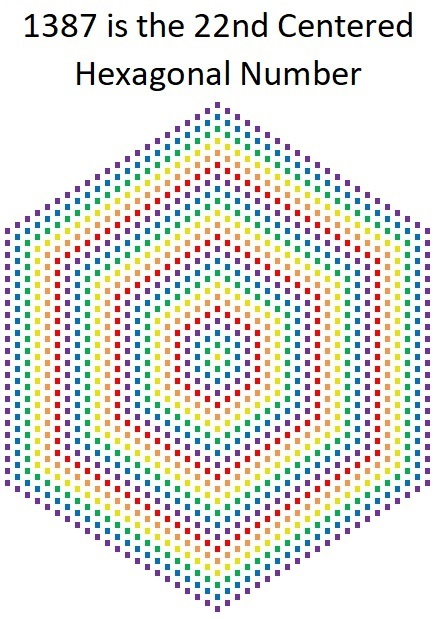# The Shape of 1387 Tiny Squares

22³ – 21³ = 1387, and that’s why it is the 22nd hexagonal number.1387 is also the 19th decagonal number. Why? Because 4(19²) – 3(19) = 1387.Here’s more about the number 1387:

• 1387 is a composite number.
• Prime factorization: 1387 = 19 × 73
• 1387 has no exponents greater than 1 in its prime factorization, so √1387 cannot be simplified.
• The exponents in the prime factorization are 1, and 1. Adding one to each exponent and multiplying we get (1 + 1)(1 + 1) = 2 × 2 = 4. Therefore 1387 has exactly 4 factors.
• The factors of 1387 are outlined with their factor pair partners in the graphic below.1387 is the hypotenuse of a Pythagorean triple:
912-1045-1387 which is 19 times (48-55-73)

This site uses Akismet to reduce spam. Learn how your comment data is processed.